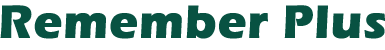5UtilitiesA renumbering utility is an important tool for the BASIC programmer. You will find "Renumber Plus" to be an invaluable aid.

When you type a BASIC statement and press RETURN, BASIC converts your code into tokens. For example, all keywords and variables become one-byte tokens. A string becomes a sequence of tokens. The first byte of the sequence—always the decimal number 15—tells BASIC that a string follows. The second byte tells BASIC the length of the string in bytes. The string appears as ASCII text following these first two bytes. When writing a program that deals with BASIC's internal form, you need to consider the format of strings to avoid problems or bugs.

The original "Renumber" by Manny Juan renumbers BASIC statements in RAM, resolves most line number references, and stays in memory for reuse.

"Renumber Plus" is a BASIC utility that enhances Manny Juan's Renumber. Renumber Plus does the following four operations the original Renumber does not:

• Resolves literal line number references after the LIST command.
• By-passes strings embedded in a statement.
• Resolves literal references following symbolic ones in a list of references.
• Allows you to choose where renumbering begins. These features add much to an already effective and useful tool.

Using Renumber Plus

1. Type Renumber Plus into your Atari.
2. Save the program with the direct command LIST "C" or LIST "D:REN". Using the LIST command allows you to merge Renumber Plus with your program.
3. LOAD your program into the Atari. The highest line number must be less than 32100. The last statement must be END, STOP or RETURN. LOADing your program erases Renumber Plus from memory.
4. Enter Renumber Plus into the Atari with the direct command ENTER"C:" or ENTER "D:REN".
5. Type GOTO 32100.
6. When the prompt BEGIN,FROM,BY appears, enter the following:
1. Beginning line number,
2. New starting line number, and
3. Increment value.
7. Enjoy the musical interlude while your Atari works. Do not press BREAK or RESET while the program renumbers. The new line number followed by SR is displayed for each symbolic reference in your program. The new line number followed by NR is displayed for each reference to an old line number that does not exist.
8. When Renumber Plus finishes renumbering, the number of renumbered lines and the following message are displayed:
`LIST "C:",bbbb,eeee`
bbbb=the first new line number
eeee=the last new line number
9. In order to save a copy of your renumbered program without the Renumber Plus program appended to it, use the LIST command (LIST "C:",bbbb,eeee for cassette and LIST "D:filename",bbbb,eeee for disk).

Renumber Plus
```32100 REM RENUMBER PLUS
32110 T8=256:I=1:Z=32100
32120 WM=0:X=PEEK(138)+PEEK(139)*T8:Y=PEEK(134)+PEEK(135)*T8+8*(PEEK(X+5)-128)+2
32130 ? "BEGIN,FROM,BY":INPUT ST,FR,BY:? CHR\$(125)
32140 B=PEEK(136)+PEEK(137)*T8:X=B:M=FR
32142 LN=PEEK(X)+PEEK(X+I)*T8
32144 IF ST>FR AND LN-ST THEN ST=LN
32150 LN=PEEK(X)+PEEK(X+I)*T8:SOUND 0,LN,10,8
32160 IF LN=Z THEN 32220
32170 PL=PEEK(X+2):C=X+3
32180 LL=PEEK(C):C=C+I
32190 GOSUB 32280
32200 IF LL<PL THEN C=X+LL:GOTO 32180
32210 X=X+PL:M=M+BY*(LN>=ST):GOTO 32150
32220 M=FR:X=B:SOUND 1,0,0,0
32230 LN=PEEK(X)+PEEK(X+I)*T8:SOUND 0,-LN+32768,10,8
32240 IF LN=Z THEN 32550
32245 IF LN<ST THEN 32270
32250 MH=INT(M/T8):ML=M-MH*T8
32260 POKE X,ML:POKE X+I,MH
32270 M=M+BY*(LN>=ST):X=X+PEEK(X+2):GOTO 32230
32280 TK=PEEK(C)
32290 IF TK=10 OR TK=11 OR TK=12 OR TK=13 OR TK=35 THEN C=C+I:GOSUB 32450:RETURN
32300 IF TK<>30 THEN 32345
32310 C=C+I:D=PEEK(C)
32320 IF D=23 OR D=24 THEN 32350
32330 IF D=14 THEN C=C+6
32335 IF D=15 THEN C=C+PEEK(C+I)+I
32340 GOTO 32310
32345 IF TK<>4 THEN 32380
32350 C=C+I:GOSUB 32450
32355 D=PEEK(C)
32360 IF D=18 THEN 32350
32362 IF D=14 THEN C=C+6
32364 IF D=15 THEN C=C+PEEK(C+I)+I
32366 IF D<>20 AND D<>22 THEN C=C+I:GO TO 32355
32370 RETURN
32380 IF TK<>7 THEN RETURN
32390 C=C+I:D=PEEK(C)
32400 IF D=27 THEN 32430
32410 IF D=14 THEN C=C+6
32415 IF D=15 THEN C=C+PEEK(C+I)+I
32420 GOTO 32390
32430 C=C+I:IF C<(X+LL) THEN GOSUB 32450
32440 RETURN
32450 D=PEEK(C):IF D=20 OR D=22 THEN C=C+I:RETURN
32460 IF D<>14 THEN ? M;" SR,";:C=C+I:RETURN
32465 DD=PEEK(C+7):IF DD<>18 AND DD<>20 AND DD<>22 THEN ? M;" SR,";:C=C+I:RETURN
32470 C=C+I:FOR J=0 TO 3:POKE Y+J,PEEK(C+J):NEXT J
32480 IF WM<LN THEN WX=B:RN=FR:GOTO 32500
32490 WX=X:RN=M
32500 WN=PEEK(WX)+PEEK(WX+I)*T8:SOUND 1,WN,10,8
32510 IF WN<Z AND WN<WM THEN RN=RN+BY*(WN>=ST):WX=WX+PEEK(WX+2):GOTO 32500
32520 IF WN<>WM THEN ? M;" NF,";:GO TO 32540
32525 IF WN<ST THEN 32540
32530 WM=RN:FOR J=0 TO 3:POKE C+J,PEEK(Y+J):NEXT J
32540 C=C+6:RETURN
32550 ? :? (M-FR)/BY;" LINES"
32560 ? "LIST";CHR\$(34);"C:";CHR\$(34);",";FR;",";M-BY
32570 END
```

Listing. Renumber Plus.